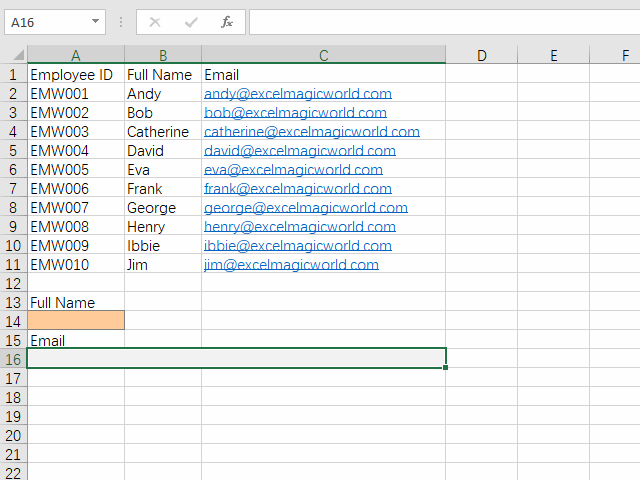top of page
Search

# How to VLookup Values from Left Columns?

Updated: Nov 9, 2019

VLookup is one of the handy functions in Excel. Everyone knows how to use it to search for value from right columns. In this section, you will learn the opposite that most people don't know."You have to set goals that are almost out of reach. If you set a goal that is attainable without much work or thought, you are stuck with something below your true talent and potential." - Steve Garvey

Vlookup() function works by looking in the first column of a table for the value you specify. It then looks across a specified number of columns and returns whatever value it finds there. The letter V stands for #Vertical. There is also Hlookup() for #Horizontal search which will be discussed in other sections.

Vlookup(lookup_value, table_array, col_index_num[, range_lookup])

looup_value: This is the value you wanna find in the first column of table_array. For example, a number, text or reference can be entered.

table_array: This is the table to use for the lookup. You can use a range reference or a range name. It's critical to determine the lookup direction. You will see the examples in this section.

col_index_num: If Vlookup() finds a match, col_index_num is the column number in table_array that contains the data you want returned.

range_lookup: This is a Boolean value that determines how Excel searches for lookup_value in the first column. If you set it as True or 1, it searches for the first exact match for lookup_value. If no exact match is found, the function looks for the largest value that is less than lookup_value. If you set it as False or 0, it searches only for the first exact match for lookup_value. This is widely used in Vlookup().

Now let us demonstrate Vlookup() with examples. Before, we will build an simple Employee table.Example 1: If the full name of an employee is given, then finds the email address.

=VLOOKUP(A14,B2:C11,2,FALSE)Example 2: If the full name of an employee is given, then finds the employee ID. Most people will copy the data of Employee ID from Column A to Column D. Actually it's not necessary. We can use Choose() function to build a new table whose first column is Full Name and second column is Employee ID.

CHOOSE({1,2},B2:B11,A2:A11)

The number 1 in the curly brackets {} stands for the first column of a table which is B2:B11 in this example.

The number 2 in the curly brackets {} stands for the second column of a table which is A2:A11.

The returned value of above Choose() function becomes the table_array of Vlookup() function. With this, you can now lookup the value from the left columns. Cool?